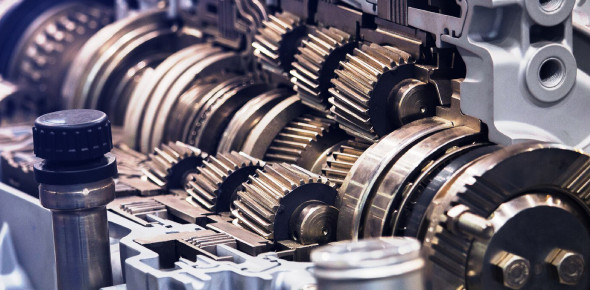How Well You Know About Gears? Trivia Quiz

8 Questions | Total Attempts: 1670SettingsHow well do you know about gears? During this quiz, you will need to know the difference between a spur gear, a helical gear, bevel gear, and worm gear, how to use a diagram to calculate the gear ratio of the compound gear train, how to calculate a speed ratio, and what is an algorithm. Get in gear and take this quiz.

• 1.
What type of gear is illustrated here?
• A.

Spur Gear

• B.

Helical Gear

• C.

Bevel Gear

• D.

Rack and Pinion

• E.

Worm Gear

• F.

Hypoid Gear

• 2.
What type of gear is illustrated here?
• A.

Spur Gear

• B.

Helical Gear

• C.

Bevel Gear

• D.

Rack and Pinion

• E.

Worm Gear

• F.

Hypoid Gear

• 3.
What type of gear is illustrated here?
• A.

Spur Gear

• B.

Helical Gear

• C.

Bevel Gear

• D.

Rack and Pinion

• E.

Worm Gear

• F.

Hypoid Gear

• 4.
What type of gear is illustrated here?
• A.

Spur Gear

• B.

Helical Gear

• C.

Bevel Gear

• D.

Rack and Pinion

• E.

Worm Gear

• F.

Hypoid Gear

• 5.
Use the diagram to calculate the gear ratio of the compound gear train.T=TeethTa=20; Tb=50; Td=75;Te=26; Tf=65
• A.

25:1

• B.

16:1

• C.

20:1

• D.

7:1

• 6.
An idler gear is a gear that will remain still in a gear train while other gears are engaged and turn.
• A.

True

• B.

False

• 7.
Check all that can be used in calculating a speed ratio for a gear train?
• A.

Length of teeth of drivers and length of teeth of drivens.

• B.

• C.

Teeth of drivers and teeth of drivens

• D.

Rotations of drivers (deg) and rotations of drivens (deg)

• E.

Speed of drivers (rpm) and speed of drivens (rpm).

• 8.
Check the boxes to the left of all the options that refer to an initial steps of a programmer in the development a working piece of code.
• A.

Algorithm

• B.

Pseudocode

• C.

Code

• D.

Flow Chart

• E.

Process Analysis

• F.

Identify Variables

Related TopicsBack to top